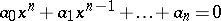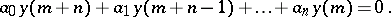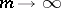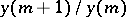# Bernoulli method

(diff) ← Older revision | Latest revision (diff) | Newer revision → (diff)
Jump to: navigation, search

A method for finding the real root of algebraic equations of the type(*)

with the largest modulus (absolute value). The method was proposed by D. Bernoulli  and is based on the following principle. Letbe random numbers and let the values ofbe calculated by the following difference equation:In general, as, the expressiontends to the value of the root of equation (*) with the largest modulus.

How to Cite This Entry:
Bernoulli method. Encyclopedia of Mathematics. URL: http://encyclopediaofmath.org/index.php?title=Bernoulli_method&oldid=12883
This article was adapted from an original article by L.N. Dovbysh (originator), which appeared in Encyclopedia of Mathematics - ISBN 1402006098. See original article